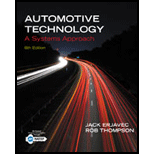Chapter 38, Problem 14RQAutomotive Technology: A Systems A...

6th Edition
Jack Erjavec + 1 other
ISBN: 9781133612315

Solutions

Chapter
SectionAutomotive Technology: A Systems A...

6th Edition
Jack Erjavec + 1 other
ISBN: 9781133612315
Textbook Problem

Which of the following gear ratios provides the highest torque multiplication?a. 0.85:1b. 2.67:1c. 5.23:1d. 0.50:1

To determine

The highest torque multiplication is provided by which gear ratio.

Explanation

Gear ratio is defined as ratio of the total number of times the output shaft turns while the input shaft is turning once. The ratio is obtained by dividing the number of teeth on the driven gear by the teeth of driving gear. The gear provides a choice between speed and torque...

Still sussing out bartleby?

Check out a sample textbook solution.

See a sample solution

The Solution to Your Study Problems

Bartleby provides explanations to thousands of textbook problems written by our experts, many with advanced degrees!

Get Started